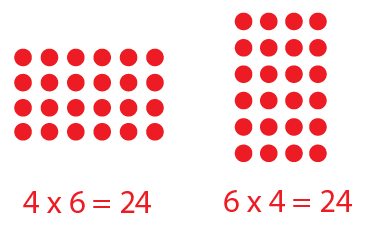3 years ago
mrs. casteel
Save
Edit
Host a game
Live GameLive
Homework
Solo Practice
Practice15 QuestionsShow answers
• Question 1
120 secondsQ. What multiplication fact goes along with this array
5 x 5
4 x 5
4 x 4
5 x 3
• Question 2
120 secondsQ. What repeated addition goes along with this array
5+5+5+5+5+5+5+5
5+5+5+5
4+4+4+4+4+4+4
4+4+4
• Question 3
120 seconds
Q. What multiplication sentence matches 4+4+4+4+4?
4 x 5
4 x 4
20
4 x 6
• Question 4
120 secondsQ.

What array is this?

5 + 5 + 5 + 5 = 20

5 + 4 = 9

4 + 4 + 4 = 20

4 + 5 = 9

• Question 5
120 secondsQ. What array is this?
2 X 5 = 10
3 X 3 = 9
2 X 4 = 8
4+4+4 = 12
• Question 6
120 secondsQ. What array is this?
4 X 3 = 12
5 X 5 = 25
4 X 3 = 16
4 X 3 = 7
• Question 7
120 secondsQ. What array is this?
4 X 8 =32
4 X 4 =16
3 X 3 = 9
4 X 5 = 20
• Question 8
300 secondsQ. What repeated addition matches this?
8+8+8+8+8 = 40
8+8+8+8+8+8 = 48
8+8+8 = 24
8+8 = 16
• Question 9
180 secondsQ. Which equation can be modeled with the following array?
3 x 12 = 4
4 x 12 = 3
4 x 3 = 12
12 x 3 = 3
• Question 10
180 secondsQ. Select the correct number model with the rectangular array:
3+3+3+3=12
4+4+4=12
3+3+3=9
4+4+4+4=16
• Question 11
180 secondsQ. Select the correct number model with the rectangular array:
2+2+2+2=8
3+3+3+3+3=15
5+5+5+5+5=25
4+4+4+4+4=20
• Question 12
180 secondsQ. Select the correct number model with the rectangular array:
4+4+4+4=16
5+5+5+5+5=25
4+4+4+4+4=20
5+5+5+5=20
• Question 13
180 secondsQ. Select the correct number model with the rectangular array:
4+4+4+4=16
2+2+2+2=8
5+5+5+5=20
3+3+3+3=12
• Question 14
180 secondsQ. Select the correct number model with the rectangular array:
4+4+4+4=16
2+2+2+2=8
2+2=4
4+4=8
• Question 15
180 secondsQ. Select the correct number model with the rectangular array